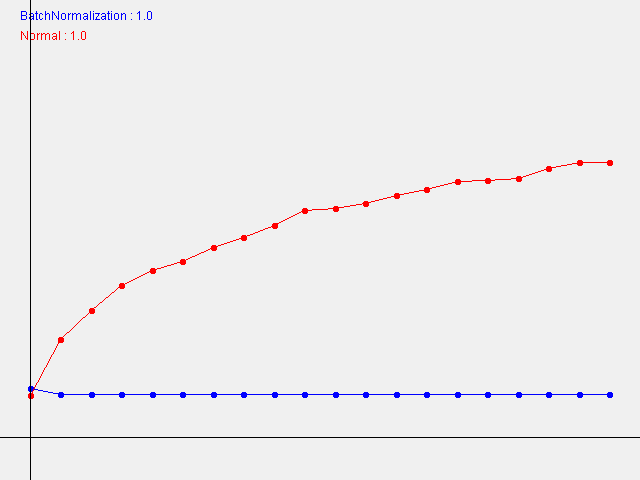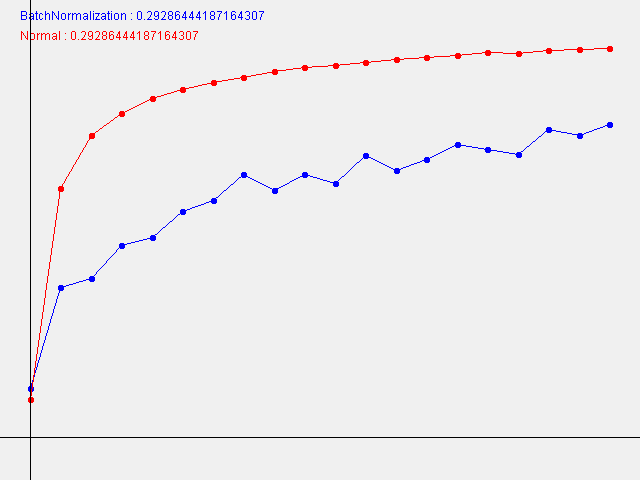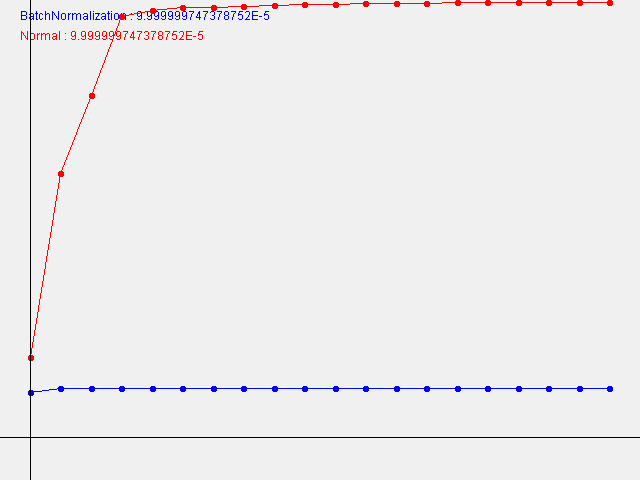# Deep Learning Java from scratch 6.3 Batch Normalization

## 6.3 Evaluation of Batch Normalization

Layer Extended to be able to pass train_flg to the interface BatchNormLayer has been created.

#### `Layer.java`

``````
public interface Layer {

INDArray forward(INDArray x);
INDArray backward(INDArray x);

}
``````

#### `BatchNormLayer.java`

``````
public interface BatchNormLayer extends Layer {

public default INDArray forward(INDArray x) {
throw new IllegalAccessError();
}

INDArray forward(INDArray x, boolean train_flg);

}
``````

The rest is the implementation of this interface Dropout class and Batch Normalization Implemented the class. In addition, implement the MultiLayerNetExtend class and execute the following test code. To do.

``````INDArray x_train;
INDArray t_train;
int max_epochs = 20;
int train_size;
int batch_size = 100;
double learning_rate = 0.01;
DataSet trainDataSet;

List<List<Double>> __train(String weight_init_std) {
MultiLayerNetExtend bn_network = new MultiLayerNetExtend(
784, new int[] {100, 100, 100, 100, 100}, 10,
/*activation=*/"relu",
/*weight_init_std=*/ weight_init_std,
/*weight_decay_lambda=*/ 0,
/*use_dropout=*/ false,
/*dropout_ration=*/ 0.5,
/*use_batchNorm=*/ true);
MultiLayerNetExtend network = new MultiLayerNetExtend(
784, new int[] {100, 100, 100, 100, 100}, 10,
/*activation=*/"relu",
/*weight_init_std=*/ weight_init_std,
/*weight_decay_lambda=*/ 0,
/*use_dropout=*/ false,
/*dropout_ration=*/ 0.5,
/*use_batchNorm=*/ false);
List<MultiLayerNetExtend> networks = Arrays.asList(bn_network, network);
Optimizer optimizer = new SGD(learning_rate);
List<Double> train_acc_list = new ArrayList<>();
List<Double> bn_train_acc_lsit = new ArrayList<>();
int iter_per_epoch = Math.max(train_size / batch_size, 1);
int epoch_cnt = 0;
for (int i = 0; i < 1000000000; ++i) {
DataSet sample = trainDataSet.sample(batch_size);
INDArray x_batch = sample.getFeatureMatrix();
INDArray t_batch = sample.getLabels();
for (MultiLayerNetExtend _network : networks) {
}
if (i % iter_per_epoch == 0) {
double train_acc = network.accuracy(x_train, t_train);
double bn_train_acc = bn_network.accuracy(x_train, t_train);
System.out.println("epoch:" + epoch_cnt + " | " + train_acc + " - " + bn_train_acc);
++epoch_cnt;
if (epoch_cnt >= max_epochs)
break;
}
}
return Arrays.asList(train_acc_list, bn_train_acc_lsit);
}

@Test
public void C6_3_2_Batch_Evaluation of Normalization() throws IOException {
// ch06/batch_norm_test.Java version of py.
MNISTImages train = new MNISTImages(Constants.TrainImages, Constants.TrainLabels);
x_train = train.normalizedImages();
t_train = train.oneHotLabels();
trainDataSet = new DataSet(x_train, t_train);
train_size = x_train.size(0);

//Drawing a graph
File dir = Constants.WeightImages;
if (!dir.exists()) dir.mkdirs();
String[] names = {"BatchNormalization", "Normal"};
Color[] colors = {Color.BLUE, Color.RED};
INDArray weight_scale_list = Functions.logspace(0, -4, 16);
INDArray x = Functions.arrange(max_epochs);
for (int i = 0; i < weight_scale_list.length(); ++i) {
System.out.println( "============== " + (i+1) + "/16" + " ==============");
double w = weight_scale_list.getDouble(i);
List<List<Double>> acc_list = __train(String.valueOf(w));
GraphImage graph = new GraphImage(640, 480, -1, -0.1, 20, 1.0);
for (int j = 0; j < names.length; ++j) {
graph.color(colors[j]);
graph.textInt(names[j] + " : " + w, 20, 20 * j + 20);
graph.plot(0, acc_list.get(j).get(0));
for (int k = 1; k < acc_list.get(j).size(); ++k) {
graph.line(k - 1, acc_list.get(j).get(k - 1), k, acc_list.get(j).get(k));
graph.plot(k, acc_list.get(j).get(k));
}
}
File file = new File(dir, "BatchNormalization#" + w + ".png ");
graph.writeTo(file);
}
}
``````

The resulting graph looks like this:

W=1.0W=0.29286444187164307W=0.00009999999747378752Unlike this book, the smaller the W (standard deviation of the initial weight), the faster the learning progresses.